# Simplifying ratios

Here you will learn about simplifying ratios, including how to simplify a ratio and how to write equivalent ratios.

Students will first learn about simplifying ratios as part of ratios and proportions in 6 th grade.

## What is simplifying ratios?

Simplifying ratios is a way of using common factors to divide all the numbers in a ratio until they cannot be divided further.

A simplified ratio is equivalent to a non simplified ratio.

You can use bar modeling to simplify ratios.

For example,

Here are some circles.

Rearrange them into colored bars to show the number of yellow circles and the number of blue circles.

The number of yellow circles is 4, and the number of blue circles is 6. This can be written as the ratio 4:6.

Rearrange these circles into another pattern, where yellow and blue have the same number of complete rows:

The overall ratio is still 4:6, but now you have two rows that show the ratio 2:3. This means that you can split the ratio into 2 groups, and still obtain an equivalent ratio of yellow to blue.

Using bar modeling simplifies the ratio 4:6 to get 2:3.

You can also divide by the greatest common factor or \bf{GCF} to simplify a ratio.

For example,

The ratio of red counters to blue counters is 12:16.

You can simplify the ratio by finding the greatest common factor or \bf{GCF} of each of the numbers in the ratio.

Factors of 12: 1, \, 2, \, 3, \, 4, \, 6, \, 12

Factor of 16: 1, \, 2, \, 4, \, 8, \, 16

The greatest common factor is 4. To simplify the ratio, you divide both sides by 4.

### What is simplifying ratios?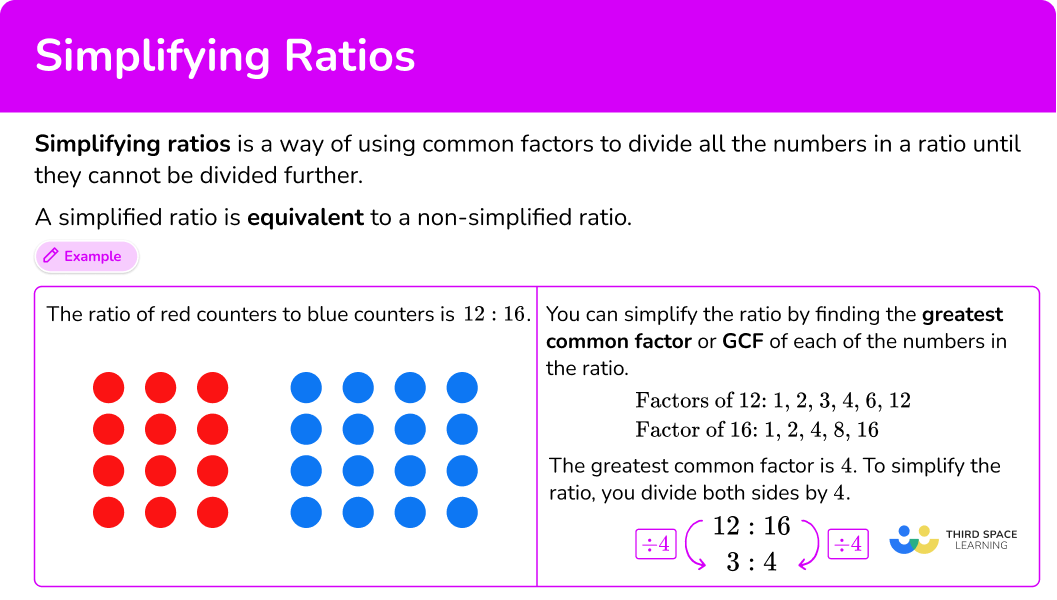## Common Core State Standards

How does this relate to 6 th grade math?

• Grade 6 – Ratios and Proportional Relationships (6.RP.A.3)
Use ratio and rate reasoning to solve real-world and mathematical problems, for example, by reasoning about tables of equivalent ratios, tape diagrams, double number line diagrams, or equations.

## How to simplify a ratio

In order to simplify a ratio:

1. Calculate the greatest common factor of the parts of the ratio.
2. Divide each part of the ratio by the greatest common factor.

## Simplifying ratios examples

### Example 1: bar modeling

Use a bar model to simplify the ratio 8:10.

1. Calculate the greatest common factor of the parts of the ratio.

Representing the ratio 8:10 as a bar model, you have…

Where red represents 8 shares and purple represents 10 shares. Thinking about how both 8 and 10 can be grouped equally, they are both divisible by 2 \, - which is also the GCF.

2Divide each part of the ratio by the greatest common factor.

Divide the bar above into two groups with the same amount of red shares and purple shares.

Each bar is the ratio 4:5, which is 8:10 in lowest terms.

### Example 2: simplifying ratios

Write the ratio 20:32 in lowest terms.

Factors of 20 = 1, \, 2, \, 4, \, 5, \, 10, \, 20

Factors of 32 = 1, \, 2, \, 4, \, 8, \, 16, \, 32

GCF (20,32) = 4

5:8 is in lowest terms.

### Example 3: simplifying ratios

Write the ratio 21:14 in lowest terms.

Factors of 21 = 1, \, 3, \, 7, \, 21

Factors of 14 = 1, \, 2, \, 7, \, 14

GCF (21,14) = 7

3:2 is in lowest terms.

### Example 4: simplifying ratios

Write the ratio 16:24 in lowest terms.

Factors of 16 = 1, \, 2, \, 4, \, 8, \, 16

Factors of 24 = 1, \, 2, \, 3, \, 4, \, 6, \, 8, \, 12, \, 24

GCF (16, 24) = 8

2:3 is in lowest terms.

### Example 5: simplifying ratios

Write the ratio 55:95 in lowest terms.

Factors of 55 = 1, \, 5, \, 11, \, 55

Factors of 95 = 1, \, 5, \, 19, \, 95

GCF (55,95) = 5

11:19 is in lowest terms.

### Example 6: simplifying ratios

Write the ratio 145:212 in lowest terms.

Factors of 145 = 1, \, 5, \, 29, \, 145

Factors of 212 = 1, \, 2, \, 4, \, 53, \, 106, \, 212

GCF (145,212) = 1

Dividing each side by 1 will not change the ratio. The ratio is already in lowest terms.

### Teaching tips for simplifying ratios

• Writing ratios is a prerequisite skill for simplifying ratios. Give students plenty of practice writing ratios from the real world, before asking them to simplify them.

• Simplifying ratios worksheets can be useful, but be sure they involve a mixture of question types, including word problems that encourage ratio problem solving.

• Let struggling students use a ratio calculator. Ask them to draw bar models of the equivalent ratios and explain how the drawings prove equivalency. Once they have enough practice doing this, they can begin solving problems on their own.

### Easy mistakes to make

• Not fully simplifying the ratio
Ratios can be simplified by other common factors, but will only be in simplest form when divided by the GCF.
For example,
Simplify the ratio 12:18.
You can simplify by dividing parts of the ratio by dividing both numbers by 2 :

While this is an equivalent ratio, this is not the lowest terms, since it can be simplified further by dividing by 3 to get the ratio 2:3.

• Thinking a ratio with smaller numbers is not equivalent
Simplifying the ratio does not change the relationship – it just shows it in a different way.
For example,

The three models of 1:3 have the same number of blue and yellow in total as the 3:9 model. That is because these two ratios are equivalent.

• Simplifying just one side of the ratio
A ratio shows the relationship between two or more numbers, so when simplifying, you must simplify all parts of the ratio.

• Using subtraction to simplify a ratio
A ratio represents a multiplicative relationship. To make a ratio smaller, divide both sides by the same number. Subtracting the same number from both sides will not keep the relationship equivalent.

• Simplifying a ratio to include decimal numbers
A simplified ratio should not include decimals.
For example,

While 0.75:1 is an equivalent ratio, when simplifying a ratio, your solution should have only whole numbers (integers). The ratio in its lowest terms is 3:4.

### Practice simplifying ratios questions

1) Which bar model shows the ratio 6:10 in lowest terms?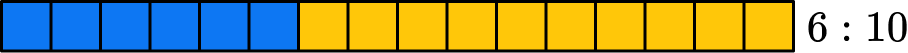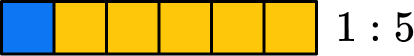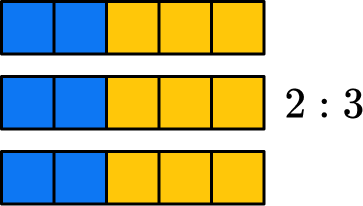The original ratio 6:10 is shown with 6 blue squares and 10 orange squares.

The greatest common factor is 2, so divide the bar above into two groups with the same amount of blue shares and orange shares.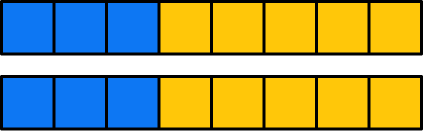Each bar is the ratio 3:5, which is 6:10 in lowest terms.

2) Write the ratio 72:24 in lowest terms.

17:414:1136:123:1Factors of 24 = 1, \, 2, \, 3, \, 4, \, 6, \, 8, \, 12, \, 24

Factors of 72 = 1, \, 2, \, 3, \, 4, \, 6, \, 8, \, 9, \, 12, \, 18, \, 24, \, 36, \, 72

GCF (24,72) = 243) Write the ratio 36:30 in lowest terms.

6:55:612:1013:15Factors of 36 = 1, \, 2, \, 3, \, 4, \, 6, \, 9, \, 12, \, 18, \, 36

Factors of 30 = 1, \, 2, \, 3, \, 5, \, 6, \, 10, \, 15, \, 30

GCF (36,30) = 64) Write the ratio 28:42 in lowest terms.

4:61:1414:212:3Factors of 28 = 1, \, 2, \, 4, \, 7, \, 14, \, 28

Factors of 42 = 1, \, 2, \, 3, \, 6, \, 7, \, 14, \, 21, \, 42

GCF (28,42) = 145) Write the ratio 78:91 in lowest terms.

78:916:713:141:3Factors of 78 = 1, \, 2, \, 3, \, 6, \, 13, \, 26, \, 39, \, 78

Factors of 91 = 1, \, 7, \, 13, \, 91

GCF (78,91) = 136) Write the ratio 102:81 in lowest terms.

102:8151:4134:274:5Factors of 102 = 1, \, 2, \, 3, \, 6, \, 17, \, 34, \, 51, \, 102

Factors of 81 = 1, \, 3, \, 9, \, 27, \, 81

GCF(102,81) = 3## Simplifying ratios FAQs

Can you write a ratio as a fraction?

Yes, you can write one part of the ratio as the numerator and the other part as the denominator.

What is the highest common factor?

This is another name for the greatest common factor (GCF).

Can parts of a ratio be something besides a whole number?

Yes, part of a ratio can be a fraction, decimal, mixed number, percentage, or other types of numbers. It can also be algebraic and show a relationship represented by polynomials.

## Still stuck?

At Third Space Learning, we specialize in helping teachers and school leaders to provide personalized math support for more of their students through high-quality, online one-on-one math tutoring delivered by subject experts.

Each week, our tutors support thousands of students who are at risk of not meeting their grade-level expectations, and help accelerate their progress and boost their confidence.